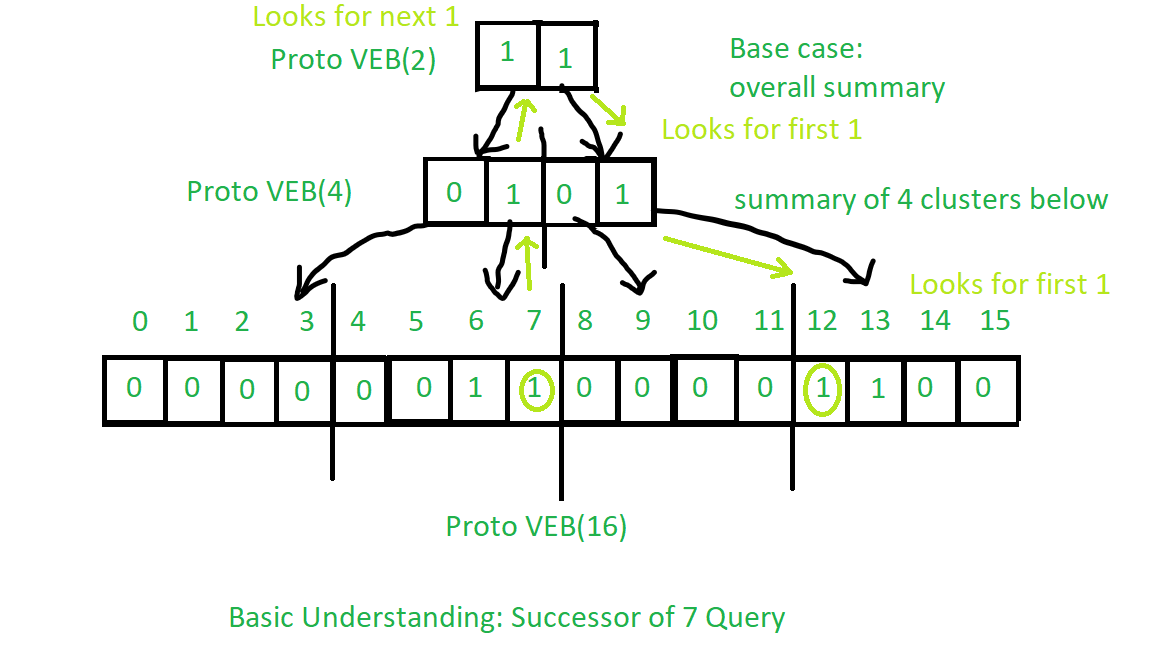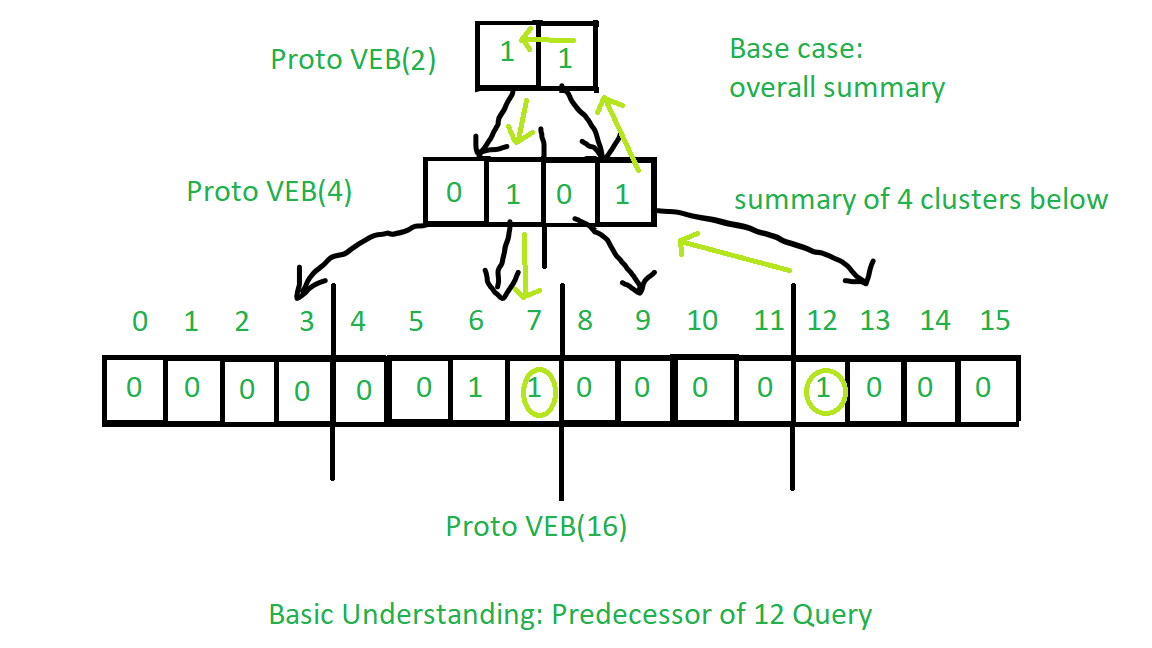# Proto Van Emde Boas Tree | Set 6 | Query : Successor and Predecessor

• Last Updated : 04 Oct, 2021

Please refer all previous articles on Proto Van Emde Boas Tree first.
Successor Query Procedure:

1. Base case: For Proto-VEB of size 2 the only possibility is that key is 0 and if the next key is present then it is its successor or there is no successor. So the same procedure is applied.
2. Recursion:
• First, we will look in the present cluster (means the cluster in which the query key is present) if there is any key greater than query key is present then we will be the successor so we return it.
• If above is not the case then we will recursively call successor procedure over summary to find next true value in summary. If there is no next true value in summary then we will return -1 as a sign that no larger key is present.
• In the above operation if we find any next true value then we will find the minimum key present in that cluster which will be the successor of the query key.

See the image below for basic understanding of the operation of Successor query:

Attention reader! Don’t stop learning now. Get hold of all the important DSA concepts with the DSA Self Paced Course at a student-friendly price and become industry ready.  To complete your preparation from learning a language to DS Algo and many more,  please refer Complete Interview Preparation Course.

In case you wish to attend live classes with experts, please refer DSA Live Classes for Working Professionals and Competitive Programming Live for Students.Procedure for Predecessor is same as successor with some minor changes you should try to understand it from the above description for successor query. See the image below for basic understanding:Below is the implementation:

## C++

 // C++ implementation of the approach#include using namespace std; class Proto_Van_Emde_Boas {public:    // Total number of keys    int universe_size;     // Summary    Proto_Van_Emde_Boas* summary;     // Clusters array of Proto-VEB pointers    vector clusters;     int root(int u)    {        return (int)sqrt(u);    }     // Function to return cluster numbers    // in which key is present    int high(int x)    {        return x / root(universe_size);    }     // Function to return position of x in cluster    int low(int x)    {        return x % root(universe_size);    }     // Function to return the index from    // cluster number and position    int generate_index(int cluster, int position)    {        return cluster * root(universe_size) + position;    }     // Constructor    Proto_Van_Emde_Boas(int size)    {        universe_size = size;         // Base case        if (size <= 2) {             // Set summary to nullptr as there is no            // more summary for size 2            summary = nullptr;             // Vector of two pointers            // nullptr in starting            clusters = vector(size, nullptr);        }        else {             // Assigning Proto-VEB(sqrt(u)) to summary            summary = new Proto_Van_Emde_Boas(root(size));             // Creating array of Proto-VEB Tree pointers of size sqrt(u)            // first all nullptrs are going to assign            clusters = vector(root(size), nullptr);             // Assigning Proto-VEB(sqrt(u)) to all its clusters            for (int i = 0; i < root(size); i++) {                clusters[i] = new Proto_Van_Emde_Boas(root(size));            }        }    }}; // Function that returns true if the// key is present in the treebool isMember(Proto_Van_Emde_Boas* helper, int key){     // If key is greater then universe_size then    // returns false    if (key >= helper->universe_size)        return false;     // If we reach at base case    // the just return whether    // pointer is nullptr then false    // else return true    if (helper->universe_size == 2) {        return helper->clusters[key];    }    else {         // Recursively go deep into the        // level of Proto-VEB tree using its        // cluster index and its position        return isMember(helper->clusters[helper->high(key)],                        helper->low(key));    }} // Function to insert a key in the treevoid insert(Proto_Van_Emde_Boas*& helper, int key){    // If we reach at base case    // then assign Proto-VEB(1) in place    // of nullptr    if (helper->universe_size == 2) {        helper->clusters[key] = new Proto_Van_Emde_Boas(1);    }    else {         // Recursively using index of cluster and its        // position in cluster        insert(helper->clusters[helper->high(key)],               helper->low(key));         // Also do the same recursion in summary VEB        insert(helper->summary, helper->high(key));    }} // Function to return the minimum key from the treeint minimum(Proto_Van_Emde_Boas* helper){    // Base case chooses the least key    // present in the cluster    if (helper->universe_size == 2) {        if (helper->clusters) {            return 0;        }        else if (helper->clusters) {            return 1;        }         // No keys present then return -1        return -1;    }    else {         // Recursively find in summary for        // first 1 present in Proto-VEB        int minimum_cluster = minimum(helper->summary);        int offset;         // If no key is present in        // the cluster then return -1        if (minimum_cluster == -1) {            return -1;        }        else {             // Recursively find the position of the key            // in the minimum_cluster            offset = minimum(helper->clusters[minimum_cluster]);             // Returns overall index of minimum key            return helper->generate_index(minimum_cluster, offset);        }    }} // Function to return the maximum key from the treeint maximum(Proto_Van_Emde_Boas* helper){     // Return the maximum key present in    // the cluster    if (helper->universe_size == 2) {        if (helper->clusters) {            return 1;        }        else if (helper->clusters) {            return 0;        }         // Return -1 if no keys present in the        // cluster        return -1;    }    else {         // Recursively find the last 1 present        // in the summary        int maximum_cluster = maximum(helper->summary);        int offset;         // If no key is present in        // the cluster then return -1        if (maximum_cluster == -1) {            return -1;        }        else {             // Recursively find the position of the key            // in the maximum_cluster            offset = maximum(helper->clusters[maximum_cluster]);            return helper->generate_index(maximum_cluster, offset);        }    }} // Function to return the successor of key in the treeint successor(Proto_Van_Emde_Boas* helper, int key){    // Base case, returns key greater than    // our query key in the cluster if present    // else returns -1    if (helper->universe_size == 2) {        if (key == 0 && helper->clusters)            return 1;        else            return -1;    }    else {         // Check if any key is greater than query key in the cluster        int offset = successor(helper->clusters[helper->high(key)],                               helper->low(key));         // If it is present then return its index        if (offset != -1)            return helper->generate_index(helper->high(key), offset);        else {             // If no successor is present within the cluster then            // go to the summmary and find the next summary with            // key present(1) named successor_cluster            int successor_cluster = successor(helper->summary,                                              helper->high(key));             // If no next 1 in the summary then return -1            if (successor_cluster == -1)                return -1;            else {                 // Find the minimum key in the successor_cluster                offset = minimum(helper->clusters[successor_cluster]);                 // Generate its index and return                return helper->generate_index(successor_cluster, offset);            }        }    }} // Function to return the predecessor of key in the treeint predecessor(Proto_Van_Emde_Boas* helper, int key){     // Base case, find smaller key present in    // the cluster    // If present else return -1    if (helper->universe_size == 2) {        if (key == 1 && helper->clusters)            return 0;        else            return -1;    }    else {         // Check if any key is lower than query key in the cluster        int offset = predecessor(helper->clusters[helper->high(key)],                                 helper->low(key));         // If it is present then return its index        if (offset != -1)            return helper->generate_index(helper->high(key), offset);        else {             // If no predecessor is present within the cluster then            // go to the summmary and find the next summary with            // key present(1) named predecessor_cluster            int predecessor_cluster = predecessor(helper->summary,                                                  helper->high(key));             // If no next 1 in the summary then return -1            if (predecessor_cluster == -1)                return -1;            else {                 // Find the maximum key in the predecessor_cluster                offset = maximum(helper->clusters[predecessor_cluster]);                 // Generate its index and return                return helper->generate_index(predecessor_cluster, offset);            }        }    }} // Function to delete a key from the treevoid pveb_delete(Proto_Van_Emde_Boas*& helper, int key){     // Base case: If the key is present    // then make it nullptr    if (helper->universe_size == 2) {        if (helper->clusters[key]) {            delete helper->clusters[key];            helper->clusters[key] = nullptr;        }    }    else {         // Recursive delete to reach at the base case        pveb_delete(helper->clusters[helper->high(key)], helper->low(key));         bool isanyinCluster = false;         // Iterate over the cluster of keys to check whether        // any other key is present within that cluster        // If yes then we should not update summary to 0        // else update summary to 0        for (int i = helper->high(key) * helper->root(helper->universe_size);             i < (helper->high(key) + 1) * helper->root(helper->universe_size);             i++) {             // If member is present then break the loop            if (isMember(helper->clusters[helper->high(key)], i)) {                isanyinCluster = true;                break;            }        }         // If no member is present then        // update summary to zero        if (isanyinCluster == false) {            pveb_delete(helper->summary, helper->high(key));        }    }} // Driver codeint main(){    Proto_Van_Emde_Boas* hello = new Proto_Van_Emde_Boas(16);     cout << boolalpha;     insert(hello, 2);     insert(hello, 13);     insert(hello, 3);     cout << successor(hello, 3) << endl;     cout << predecessor(hello, 13) << endl;}

Recurrence Relation for Successor and Predecessor Queries:

T(u) = T(u) = 2T()) + O(log2())

Time Complexity: O(log2(u)*log2(log2(u))) per query
Auxiliary Space: O(N).

My Personal Notes arrow_drop_up